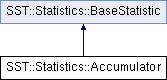SST  6.1.0 StructuralSimulationToolkit
SST::Statistics::Accumulator Class Reference

Allows the online gathering of statistical information about a single quantity. More...

`#include <accumulator.h>`

Inheritance diagram for SST::Statistics::Accumulator:## Public Member Functions

Accumulator (char *name)
Create a new Accumulator class with initial values set to a zero count, zero sum statistic of interest.

NumberBase getSum ()
Provides the sum of the values presented so far. More...

NumberBase getSumSquared ()
Provides the sum of each value squared presented to the class so far. More...

Present a new value to the class to be included in the statistics. More...

void add (NumberBase *values, uint32_t length)
Present an array of values to the class to be included in the statistics. More...

NumberBase getArithmeticMean ()
Get the arithmetic mean of the values presented so far. More...

NumberBase getVariance ()
Get the variance of the values presented so far. More...

NumberBase getStandardDeviation ()
Get the standard deviation of the values presented so far. More...

uint64_t getCount ()
Get a count of the number of elements presented to the statistics collection so far. More...Public Member Functions inherited from SST::Statistics::BaseStatistic
BaseStatistic (std::string statName)
Constructor for the BaseStatistics class. More...

BaseStatistic (char *statName)
Constructor for the BaseStatistic class. More...

const char * getName ()
Get the name of the statistic. More...

void enable ()
Enable the statistic for collection.

void disable ()
Disable the statistic collection.

bool isEnabled ()
Query whether the statistic is currently enabled. More...Protected Attributes inherited from SST::Statistics::BaseStatistic
char * name

bool enabled

## Detailed Description

Allows the online gathering of statistical information about a single quantity.

The basic statistics are captured online removing the need to keep a copy of the values of interest.

Template Parameters
 NumberBase A template for the basic numerical type of values

## Member Function Documentation

 void SST::Statistics::Accumulator::add ( NumberBase value )
inline

Present a new value to the class to be included in the statistics.

Parameters
 value New value to be presented
 void SST::Statistics::Accumulator::add ( NumberBase * values, uint32_t length )
inline

Present an array of values to the class to be included in the statistics.

Parameters
 values The array of values to be added to the statistics collection length The length of the array being presented
 NumberBase SST::Statistics::Accumulator::getArithmeticMean ( )
inline

Get the arithmetic mean of the values presented so far.

Returns
The arithmetic mean of the values presented so far.
 uint64_t SST::Statistics::Accumulator::getCount ( )
inline

Get a count of the number of elements presented to the statistics collection so far.

Returns
Count the number of values presented to the class.
 NumberBase SST::Statistics::Accumulator::getStandardDeviation ( )
inline

Get the standard deviation of the values presented so far.

Returns
The standard deviation of the values presented so far

References getVariance().

 NumberBase SST::Statistics::Accumulator::getSum ( )
inline

Provides the sum of the values presented so far.

Returns
The sum of values presented to the class so far.
 NumberBase SST::Statistics::Accumulator::getSumSquared ( )
inline

Provides the sum of each value squared presented to the class so far.

Returns
The sum of squared values presented to the class so far.
 NumberBase SST::Statistics::Accumulator::getVariance ( )
inline

Get the variance of the values presented so far.

Returns
The variance of the values presented so far

Referenced by getStandardDeviation().

The documentation for this class was generated from the following file: